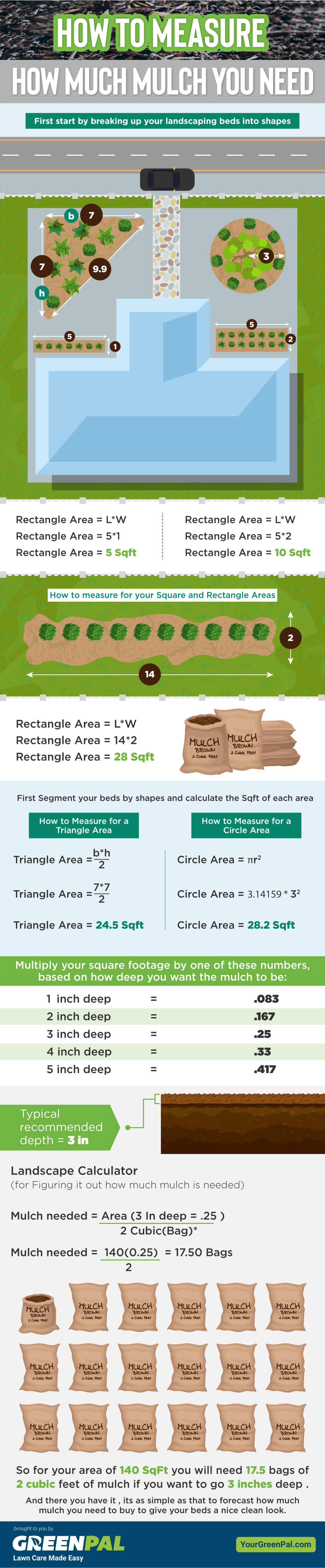### How to Measure How Much Mulch You Need (Illustrated)

• by
• Gene Caballero
• September 10, 2020

Measuring mulch can be an intimidating task. Especially for homeowners with little experience. But don’t worry, learning how to measure the amount of mulch you need is very easy, and this infographic explains EVERYTHING that you need to know.

Here are some of the most frequently asked questions about how to measure mulch for your landscaping.

### How deep should I plan to mulch?

In general 3-4 inches is adequate to get the most out of mulching your landscape. Although if you have a lot of weeds you want to choke out, you can put as much as 8 inches deep. This also depends on the material you are using as well.

### How do I measure the square footage of a triangular bed?

When you are measuring a triangular garden space, you will multiply the base by the height, and divide it by two. In this example, the base is 7 and the height is 7. 7*7 is 49. Divided by two you will need enough mulch to cover 24.5 square feet. Next you will want to calculate the depth.

### How do I calculate mulch to be as deep as I want it?

Once you have calculated the square footage of the area you want to mulch, simply multiply your square footage by the numbers on the chart in the infographic. For example, if you want mulch 100 square feet of garden space three inches deep. You will multiply 100 square feet by .25 (or 1/4 of a foot) which is 25. In this example, 25 represents the number of cubic feet you will need.

### How many cubic feet are in a bag of mulch?

Most bags of wooden mulch contain 2 cubic square feet of mulch. On the other hand, most bags of stone mulch contain around .5 cubic feet of material.

### How many cubic feet are in a yard?

There are 27 Cubic feet in one cubic yard. So if you need to purchase a cubic yard of bagged mulch, you would need 13.5 bags of mulch.

## How to Calculate Mulch in a Nutshell

It’s not difficult to calculate how much mulch you will need for your garden beds. Using the formulas in this infographic will make measuring mulch a breeze! Keep this infographic on hand for when you need it, and be sure to share it with your friends and family via email, and on social media!